# LOOKUP Function

Returns a value from a one-row or one-column range

## What is the LOOKUP Function?

The LOOKUP Function is categorized under Excel Lookup and Reference functions. The function performs a rough match lookup either in a one-row or one-column range and returns the corresponding value from another one-row or one-column range.

While doing financial analysis, if we wish to compare two rows or columns, we can use the LOOKUP function. It is designed to handle the simplest cases of vertical and horizontal lookup.

The more advanced versions of the LOOKUP function are HLOOKUP and VLOOKUP.

### Formula (Vector)

There are two forms of Lookup: Vector and Array.

The vector form of the LOOKUP function will search one row or one column of data for a specified value and then get the data from the same position in another row or column.

The formula for the function is as follows:

=LOOKUP(lookup_value, lookup_vector, [result_vector])

It uses the following arguments:

1. Lookup_value (required function) – This is the value that we will be searching. It can be a logical value of TRUE or FALSE, reference to a cell, number, or text.
2. Lookup_vector (required function) – This is the one-dimensional data that we wish to search. Remember, we need to sort it in ascending order.
3. Result_vector – An optional one-dimensional list of data from which we want to return a value. If supplied, the [result_vector] must be the same length as the lookup_vector. If the [result_vector] is omitted, the result is returned from the lookup_vector.

The array form of LOOKUP looks in the first row or column of an array for the specified value and returns a value from the same position in the last row or column of the array. We need to use this form of LOOKUP when the values that we want to match are in the first row or column of the array.

### Formula (Array) LOOKUP Function

= LOOKUP(lookup_value, array)

The arguments are as follows:

1. Lookup_value (required argument) – This is a value that we are searching for.
2. Array (required argument) – A range of cells that contains text, numbers, or logical values that we want to compare with the lookup_value.

### How to use the LOOKUP Function in Excel?

As a worksheet function, the LOOKUP Function can be entered as part of a formula in a cell of a worksheet. To understand the uses of this function, let us consider a few examples:

#### Example 1

Assume we are given a list of products, color, order_id, and quantity. We want a dashboard where we put the product and then we instantly get the quantity.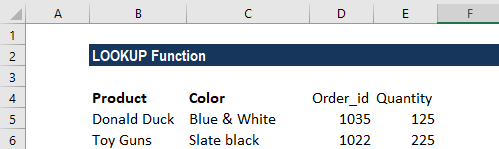The formula to use will be: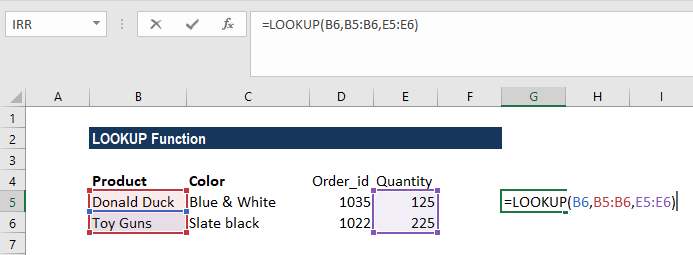The result we get is: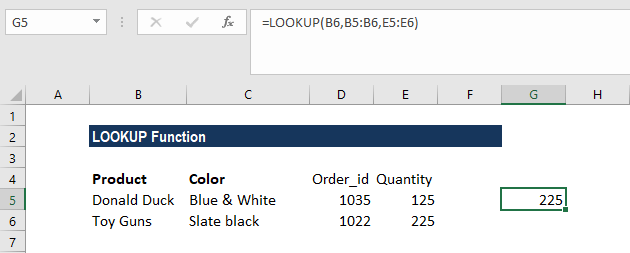#### Example 2

Suppose we are in the business of giving loans and we offer different interest rates based on the amount borrowed. We are given the data below: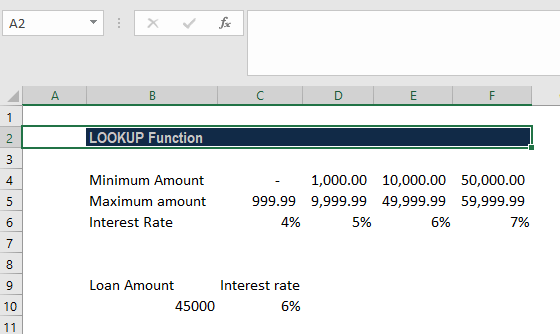The formula to use will be: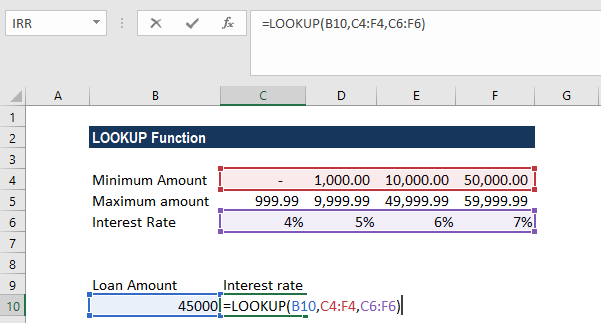We will get the following result: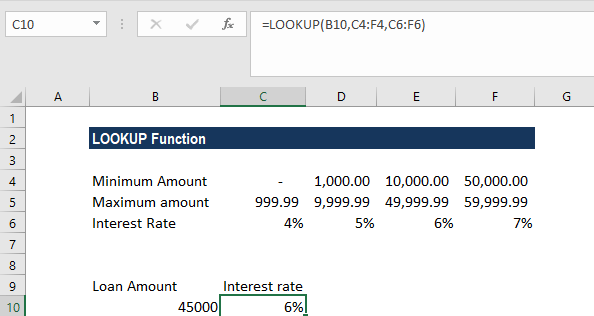### Things to remember about the LOOKUP Function:

1. #N/A error – Occurs when the Lookup function fails to find the closest match to the supplied lookup_value. This can occur if either:
1. The smallest value in the lookup_vector (or first column/row of the array) is greater than the lookup_value provided; or
2. The lookup_vector (or first column/row of the array) is not in ascending order.
2. #REF! error – Occurs if the formula is attempting to reference cells that are non-existent. This can be caused by either:
1. Cells being deleted after the Lookup function has been entered.
2. Relative references in the Lookup function that have become invalid when the functions have been copied to other cells.

Thanks for reading CFI’s guide to important Excel functions! By taking the time to learn and master these functions, you’ll significantly speed up your financial analysis. To learn more, check out these additional CFI resources:

• Excel Functions for Finance